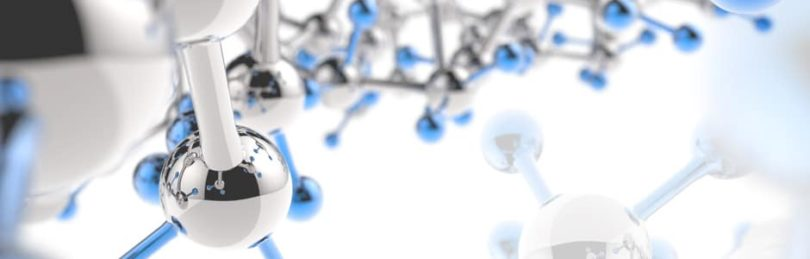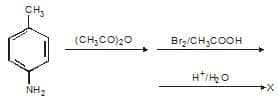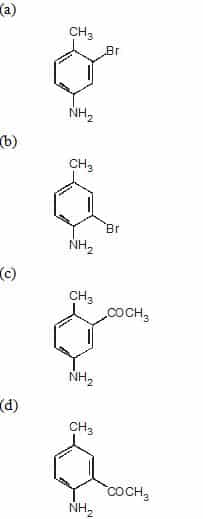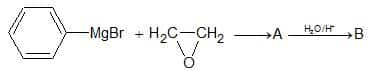# BITSAT Chemistry Sample PaperBITSAT Chemistry Sample paper consist 40 questions based on 11th and 12th Chemistry syllabus of CBSE Board.

Question: Mark the gas which turns lime water milky
(a) H2S
(b) SO2
(c) Cl2
(d) CO2

Ans. (b), (d)

Question:The product is :Ans. (b)

Question: Which is the decreasing order of stability?
(i) CH– CH – CH3
(ii) CH– CH – O – CH3
(iii) CH3CH – CO – CH3
(a) (i) < (ii) < (iii)
(b) (i) > (ii) > (iii)
(c) (iii) > (i) > (ii)
(d) (ii) > (iii) > (i)

Ans. (b)

Question: Which of the following reactions with H2S does not produce metallic sulphide
(a) ZnCl2
(b) CdCl2
(c) COCl2
(d) CuCl2

Ans. (c)

Question:The density of H2S (mol. wt. 34) at 27°C and 2 atm pressure (R = 0.0821 atm/mol K):
(a) 2.76g/L
(b) 27.6 g/L
(c) 2.76 mg/L
(d) none of these

Ans. (a)

Question:Which of the following will have the maximum dipole moment ?
(a) CH3F
(b) CH3CI
(c) CH3Br
(d) CH3I

Ans. (b)

Question: Boiling point of the following compounds follow the order.
(a) CH3CH3 <CH3NH2 <CH3OH< HCOOH
(b) CH3NH2 < CH3OH < CH3CH3 < HCOOH
(c) CH3OH < CH3CH3 < CH3NH2 < HCOOH
(d) HCOOH < CH3NH2 < CH3OH < CH3CH3

Ans. (a)

Question: Which of the following salts is colourless ?
(a) CdCI2
(b) CuSO4-5H2O
(c) MnSO4-7H2O
(d) NiSO4-7H

Ans. (a)

Question: What are the shapes of (i) C1F3 and (ii) BrF3 molecules ?
(a) Both are planar trigonal
(b) Both have distorted T-shaped
(c) (i) is planar trigonal and (ii) is T-shaped
(d) (i) is T-shaped and (ii) is planar trigonal

Ans. (b)

Question: An organic compound containing C, H and O contains 52.5% C and 13.04% Hr Vapour density of the
compound is 23. Its molecular formula will be :
(a) C2H6O
(b) C3H8O
(c) C4H8O
(d) C5H10O

Ans. (a)

Question: An ester (A) with molecular formula C9Hi0O2 was treated with excess of CH3MgBr and the compound so formed was treated with cone. H2SO4 to form olefin (B). Ozonolysis of B gave ketone with formula C8H8O. Which shows positive iodoform test the structure of A is:
(a) CH3CH2COC6CH5
(b) C6H5COOC2H5
(c) C6HSCOOC6H5
(d) CH3COC6H4COCH3

Ans. (b)

Question: The only liquid non-metal present in periodic table is:
(a) chlorine
(b) mercury
(c) bromine
(d) fluorine

Ans. (c)

Question: Which of the following is not correct for D2O ?
(a) BVP is higher than H2O
(c) Viscosity is higher than H2O at 25°
(b) D2O reacts slowly than H2O
(d) Solubility of NaCI in it is more than H2O

Ans. (d)

Question: Under critical states of a gas for one mole of a gas, compressibility factor is
(a) 3/8
(b) 8/3
(c) 1
(d) ¼

Ans. (a)

Question: Which of the following is neutral refractory material?
(a) SiO2
(b) MgO
(c) CaO
(d) SiC

Ans. (d)

Question: Number of n electrons present in naphthalene is :
(a) 4
(b) 6
(c) 10
(d) 14

Ans. (c)

Question: The low density of ice compared to water is due to:
(a) hydrogen bonding interactions
(b) dibble-dipole interactions
(c) dipole induced dipole interactions
(d) induced  dipole induced dipole interactions

Ans. (a)

Question: Galvanic cell involves:
(a) conversion of thermal energy (heating) into electrical energy
(b) conversion of electrical energy into chemical energy
(c) conversion of chemical energy into thermal energy
(d) conversion of chemical energy into electrical energy

Ans. (d)

Question: Name of the compound given below is :
(a) 3-methyl-4-ethyloctane
(b) 2, 3-diethylheptane
(c) 5-ethyl-6-methyloctane
(d) 4-ethyl-3-methyloctane

Ans. (d)

Question: A relation between vapour pressure and temperature is known as :
(a) gas equation
(b) Clapeyron equation
(c) Claussius equation
(d) Claussius-Clapeyron equation

Ans. (d)

Question: Adsorption occurs not due to:
(a) unbalanced force at surface molecules
(b) unutilized free valencies at surface
(c) increased entropy at surface
(d) van der Waals’ attraction at surface

Ans. (c)

Question: In a hydrogen atom, if the energy of an electron in the ground state is 13.6 eV, then that in the second excited state is :
(a) 1.51 eV
(b) 3.4 eV
(c) 6.04 eV
(d) 13.6 eV

Ans. (a)

Question: Borax structure contains:
(a) two BO4 groups and two BO3 groups
(b) four BO4 groups only
(c) four BO3 groups only
(d) three BO4 and one BO3 groups

Ans. (a)

Question: Dacron is copolymer of:
(a) hexamethylene diamine and adipic acid
(b) ethylene glycol and terepthalic acid
(c) ethylene and pthalic acid

Ans. (b)

Question: In the sequence of reactions,The product B is

(a) Benzyl alcohol
(b) 2-Phenyl ethanol
(c) 1-Phenyl ethanol
(d) Quinol

Ans. (b)

Question: When D-glucose is oxidized by Ag:0 gives:
(a) L-glucaric acid
(b) D-glucaric acid
(c) D-gluconic acid
(d) L-gluconic acid

Ans. (c)

Question: The order of reactivities of methyl halides in the formation of Grignard reagent is
(a) CH3I > CH3Br > CH3Cl
(b) CH3Cl > CH3Br > CH3I
(c) CH3Br > CH3Cl > CH3I
(d) CH3Br > CH3I > CH3Cl

Ans. (a)

Question: neo-pentylbromide on reaction with alc. KOH yields ______ as the major product.
(a) 2-Methyl-1-Butene
(b) 2-Methyl-2-Butene
(c) 2-2-Dimethyl-1-propanol
(d) 2-Methyl-2-Butanol

Ans. (b)

Question: Which of the following is not an electrophile
(a) Cl+
(b) Na+
(c) H+
(d) BF3

Ans. (b)

Question: The ion which exhibits green colour?
(a) Cu2+
(b) Mn2+
(c) Co2+
(d) Ni2+

Ans. (d)

Question: Identify the correct statement
(a) Gypsum contains a lower percentage of calcium than Plaster of Paris.
(b) Gypsum is obtained by heating Plaster of Paris.
(c) Plaster of Paris can be obtained by hydration of Gypsum.
(d) Plaster of Paris is obtained by partial oxidation of Gypsum.

Ans. (a)

Question: 1 mole of N2O4 (g) at 300 K is kept in a closed container under one atmosphere. It is heated to 600 K when 20% by mass of N2O4 (g) decomposes to NO2 (g). The resultant pressure is:
(a) 1.2 atm
(b) 2.4 atm
(c) 2.0 atm
(d) 1.0 atm

Ans. (b)

Question: Which destroy antigens?
(a) Insulin
(b) Antibodies
(c) Chromoprotein
(d) Phosphoprotein

Ans. (b)

Question: When Na reacts with liquid NH3 the following substance is formed:
(a) [Na(NH3)x]
(b) [e(NH3)y]
(c) NaNH2
(d) NaxNH3y

Ans. (b)

Question: Which ion has the lowest radius from the following ions
(a) Na+
(b) Mg2+
(c) Al3+
(d) Si4+

Ans. (d)

Question: Glyptal polymer is obtained by the reaction of glycerol with :
(a) Malonic acid
(b) acetic acid
(c) phthalic acid
(d) maleic acid

Ans. (c)

Question: The purine base present in RNA is :
(a) guanine
(b) thymine
(c) cytosine
(d) uracil

Ans. (d)

Question: If the H+ concentration is decreased from 1M to 10-4 M at 25o C for the couple MnO4/Mn2+, then the oxidizing power of the MnO4/Mn2+ couple decrease by :
(a) -0.18 V
(b) 0.18 V
(c) 0.38 V
(d) -0.38 V

Ans. (c)

Question: Pinacol is :
(a) 3-methylbutan-2-ol
(b) 2,3-dimethyl-2,3-butanediol
(c) 2,3-dimethyl-2-propanone
(d) none of the above

Ans. (b)

Question: The order of increasing energies of the orbitals follows:
(a) 5p < 4f < 6s < 5d
(b) 5p < 6s < 4f < 5d
(c) 4f < 5p < 5d < 6s
(d) 5p < 5d < 4f < 6s

Ans. (b)

Related: general questions in maths

Question: A hydride of nitrogen which is acidic is
(a) NH3
(b) N2H2
(c) N3H
(d) N2H4

Ans. (c)

Question: The compound with negative heat of formation is known as
(a) Endothermic compound
(b) Exothermic compound
(c) Heat of formation compound
(d) None of the above

Ans. (b)

Ques. Which is the strongest reducing agent?
(a) F
(b) Cl
(c) Br
(d) I

Ans. (d)

Ques. A gem dihalide is not the product of the following reactions
(a) Vinyl chloride + HCl
(b) Acetone + PCl5
(c) Methylacetylene + 2HBr
(d) Ethyne +2Cl2

Ans. (d)

Ques. Which of the following electrolytes is least effective in coagulating a colloidal solution of ferric hydroxide
(a) K3[Fe(CN)6]
(b) K2CrO4
(c) KBr
(d) K2SO4

Ans. (c)

Ques. Colligative properties of solutions depends on
(a) Nature of solute
(b) Nature of solvent
(c) Number of particles present in the solution
(d) Only mole number of solvent

Ans. (c)

Ques. Which of the following names is acceptable for the simplest member of the alkyl cyanide family?
(a) Methanenitrile
(b) Ethanenitrile
(c) Ethylnitrile
(d) Methylnitrile

Ans. (b)

Ques. Which of the following reactions can be used for preparing an aliphatic primary amine, but not aniline?
[A] Reduction of a nitro compound
[B] Wurtz method
[C] Reduction of an aldoxime
[D] Mendius reaction
(a) A and B
(b) B and C
(c) A and C
(d) C and D

Ans. (d)

Ques. Which of the following is formed on heating acetaldehyde in the presence of anhydrous aluminium chloride and aluminium chloride and aluminium alkoxide?
(a) An alkanoic acid
(b) Alkyl alkanoate
(c) An alkanol
(d) An aldol

Ans. (b)

Ques. Maximum number of electrons in any orbit is
(a) n2
(b) 2n2
(c) ½ n2
(d) None of these

Ans. (b)